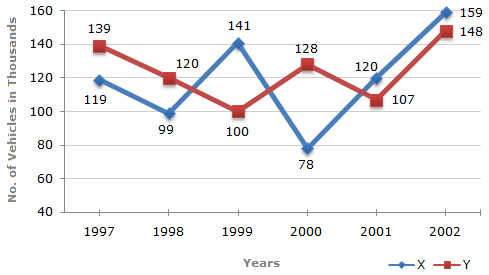# Data Interpretation - Line Charts

Exercise : Line Charts - Line Chart 3
Directions to Solve

Study the following line graph and answer the questions based on it.

Number of Vehicles Manufactured by Two companies ove the Years (Number in Thousands)1.
What is the difference between the number of vehicles manufactured by Company Y in 2000 and 2001 ?
50000
42000
33000
21000
Explanation:

Required difference = (128000 - 107000) = 21000.

2.
What is the difference between the total productions of the two Companies in the given years ?
19000
22000
26000
28000
Explanation:

From the line-graph it is clear that the productions of Company X in the years 1997, 1998, 1999, 2000, 2001 and 2002 are 119000, 99000, 141000, 78000, 120000 and 159000 and those of Company Y are 139000, 120000,100000, 128000, 107000 and 148000 respectively.

Total production of Company X from 1997 to 2002

= 119000 + 99000 + 141000 + 78000 + 120000 + 159000

= 716000.

and total production of Company Y from 1997 to 2002

= 139000 + 120000 + 100000 + 128000 + 107000 + 148000

= 742000.

Difference = (742000 - 716000) = 26000.

3.
What is the average numbers of vehicles manufactured by Company X over the given period ? (rounded off to nearest integer)
119333
113666
112778
111223
Explanation:

Average number of vehicles manufactured by Company X

 = 1 x (119000 + 99000 + 141000 + 78000 + 120000 + 159000) 6

= 119333.

4.
In which of the following years, the difference between the productions of Companies X and Y was the maximum among the given years ?
1997
1998
1999
2000
Explanation:

The difference between the productions of Companies X and Y in various years are:

For 1997 (139000 - 119000) = 20000.

For 1998 (120000 - 99000) = 21000.

For 1999 (141000 - 100000) = 41000.

For 2000 (128000 - 78000) = 50000.

For 2001 (120000 - 107000) = 13000.

For 2002 (159000 - 148000) = 11000.

Clearly, maximum difference was in 2000.

5.
The production of Company Y in 2000 was approximately what percent of the production of Company X in the same year ?
173
164
132
97
 Required percentage =128000 x 100%164%. 78000